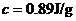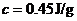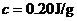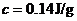Chapter 10, Problem 16ALQ### Introductory Chemistry: A Foundati...

9th Edition
Steven S. Zumdahl + 1 other
ISBN: 9781337399425

#### Solutions

Chapter
Section### Introductory Chemistry: A Foundati...

9th Edition
Steven S. Zumdahl + 1 other
ISBN: 9781337399425
Textbook Problem
68 views

# Consider four 100.0-g samples of water, each in a separate beaker at 25.0 °C. Into each beaker you drop 10.0 g of a different metal that has been healed to 95.0 °C. Assuming no heat loss to the surroundings, which water sample will have the highest final temperature? Explain your answer.l type='a'>The water to which you have added aluminum°C).i>The water to which you have added iron°C)i>The water to which you have added copper°C).i>The water to which you have added lead°C).i>Because the masses of the metals are the same, the final temperatures would be the same.

Interpretation Introduction

(a)

Interpretation:

In the given reaction, which water sample will have the highest final temperature should be determined.

Concept Introduction:

The Calorimetric expression is

Q=ms×DTs×SHs----(1)Here, Q is the heat involved, msis the mass of the sampleDTsis change in temperature and SHs is the specific heat of sample.As given ms=100.0gWe know, DTs=T2-T1T2 is the final temperature and T1=25oCSubstituting these values in the equation 1Qw=418.4×(T2-25)J/oC.

Explanation

Metal given is aluminium, mass of aluminium is 10.0 g, SHs for aluminium is 0.89J/g0 C. Assume the final temperature of the sample be T2 andT1 = 95 0 C

Substitute these values in the equation 1

QAl=8.9(T2-95)J/goC

We know that the heat lost by metal is same as heat gained by water, so we have

-QAl=Qw-8

Interpretation Introduction

(b)

Interpretation:

In the given reaction, which water sample will have the highest final temperature should be determined.

Concept Introduction:

The Calorimetric expression is

Q=ms×DTs×SHs----(1)Here, Q is the heat involved, msis the mass of the sampleDTsis change in temperature and SHs is the specific heat of sample.As given ms=100.0gWe know, DTs=T2-T1T2 is the final temperature and T1=25oCSubstituting these values in the equation 1Qw=418.4×(T2-25)J/oC.

Interpretation Introduction

(c)

Interpretation:

In the given reaction, which water sample will have the highest final temperature should be determined.

Concept Introduction:

The Calorimetric expression is

Q=ms×ΔTs×SHs----(1)Here, Q is the heat involved,  msis the mass of the sampleΔTsis change in temperature and SHs is the specific heat of sample.As given  ms=100.0gWe know,  ΔTs=T2-T1T2 is the final temperature and T1=25oCSubstituting these values in the equation 1Qw=418.4×(T2-25)J/oC.

Interpretation Introduction

(d)

Interpretation:

In the given reaction, which water sample will have the highest final temperature should be determined.

Concept Introduction:

The Calorimetric expression is

Q=ms×DTs×SHs----(1)Here, Q is the heat involved, msis the mass of the sampleDTsis change in temperature and SHs is the specific heat of sample.As given ms=100.0gWe know, DTs=T2-T1T2 is the final temperature and T1=25oCSubstituting these values in the equation 1Qw=418.4×(T2-25)J/oC.

Interpretation Introduction

(e)

Interpretation:

In the given problem if the mass of all the samples is the same then final temperatures would same or not should be determined.

Concept Introduction:

The Calorimetric expression is

Q=ms×ΔTs×SHs----(1)Here, Q is the heat involved,  msis the mass of the sampleΔTsis change in temperature and SHs is the specific heat of sample.As given  ms=100.0gWe know,  ΔTs=T2-T1T2 is the final temperature and T1=25oCSubstituting these values in the equation 1Qw=418.4×(T2-25)J/oC.

### Still sussing out bartleby?

Check out a sample textbook solution.

See a sample solution

#### The Solution to Your Study Problems

Bartleby provides explanations to thousands of textbook problems written by our experts, many with advanced degrees!

Get Started